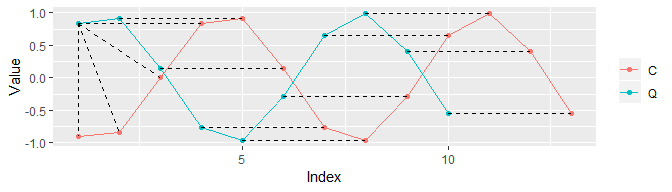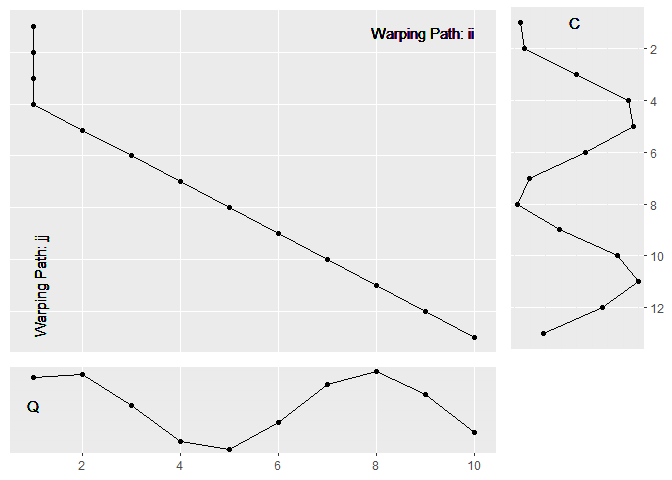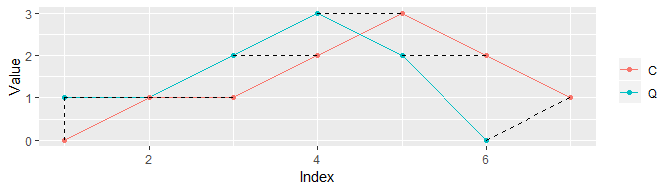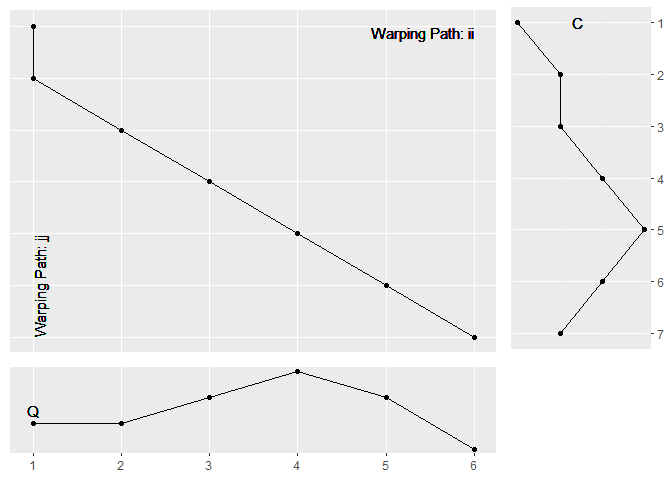# Incremental Dynamic Time Warping

#### 2019-01-02

Dynamic Time Warping is a well known and often applied technic to measure the distance between two time series. (Google) finds about 750,000 matches for the term Dynamic Time Warping. Searching the scientific search engines for research articles for this subject results in:

To learn more about DTW interested readers may also read (Wikipedia DTW) or have look at this (Dummy Example). This (tutorial) also explains the main idea of DTW, described in the original paper by (Sakoe and Chiba: Dynamic programming algorithm optimization for spoken word recognition)

Outline:

• Introduction to Dynamic Time Warping: dtw()
• Incremental Calculation of DTW: idtw()
• Calculation Time and Comparisons
• Decremental Dynamic Time Warping: dec_dm()
• Vector-based Implementation of DTW: dtw2vec()

## Introduction to Dynamic Time Warping: dtw()

The distance measure DTW calcuates the minimal costs of the shortest non-linear alignment of two time series $$Q$$ (the query time series) and $$C$$ (the candidate time series), conditional to

• The first element of $$Q$$ is aligned to the first element of $$C$$
• The last element of $$Q$$ is aligned to the last element of $$C$$
• A 1:k alignment is applied (unlike to the Euclidean distance calcuation). That is, one observation of $$Q$$ can be applied to k-many of $$C$$, and vice versa.

Remark: The DTW distance measure is not a distance metric in the conventional sense, since it does not fulfill the triangle inequality.

In the following we show by aid of toy example how to use the functions of IncDTW and what they are doing. We start with the basic functions. Suppose we have two time series and we need to calculate the DTW distance of these:

set.seed(1090)
Q <- sin(1:10)#+rnorm(20)
C <- sin(-2:10)#+rnorm(15)
tmp <- IncDTW::dtw(Q = Q, C = C, return_diffM = TRUE, return_wp = TRUE,
return_QC = TRUE, return_cm = TRUE, return_diffp = TRUE)
names(tmp)
##   "gcm"                 "dm"                  "diffp"               "ii"                  "jj"                  "wp"                  "distance"            "normalized_distance" "cm"                  "diffM"               "Q"                   "C"Another toy example:

set.seed(1090)
Q <- c(1, 1, 2, 3, 2, 0)
C <- c(0, 1, 1, 2, 3, 2, 1)

tmp <- IncDTW::dtw(Q = Q, C = C, return_diffM = TRUE, return_wp = TRUE,
return_QC = TRUE, return_cm = TRUE, return_diffp = TRUE)
names(tmp)
##   "gcm"                 "dm"                  "diffp"               "ii"                  "jj"                  "wp"                  "distance"            "normalized_distance" "cm"                  "diffM"               "Q"                   "C"The matrix of differences diffM simply stores the differences of the two time series $$Q$$ and $$C$$.

tmp$diffM ## [,1] [,2] [,3] [,4] [,5] [,6] [,7] ## [1,] 1 0 0 -1 -2 -1 0 ## [2,] 1 0 0 -1 -2 -1 0 ## [3,] 2 1 1 0 -1 0 1 ## [4,] 3 2 2 1 0 1 2 ## [5,] 2 1 1 0 -1 0 1 ## [6,] 0 -1 -1 -2 -3 -2 -1 The global cost matrix is calculated by walking through the matrix of absolute differences abs(diffM) from top left to bottom right applying the following rule: cm <- abs(diffM) # cost matrix gcm <- cm # initialize the global cost matrix for(j in 1:m){ for(i in 1:n){ gcm[ , 1] <- cm[ ,1] gcm[1, ] <- cm[1, ] gcm[i, j] <- cm[i, j] + min(c(gcm[i-1, j-1], gcm[i , j-1], gcm[i-1, j ]))}} The direction matrix dm is calculated simultaneously to the global cost matrix and stores for each position where to go next to make the cheapest step. dm <- matrix(NA, ncol=length(C), nrow = length(Q)) dm[1, ] <- 3 dm[ , 1] <- 2 dm[1, 1] <- NA for(j in 1:m){ for(i in 1:n){ min_index <- which.min(c(gcm[i-1, j-1], gcm[i , j-1], gcm[i-1, j ])) if( min_index == 1){ dm[i, j] <- 1 } else if( min_index == 2){ dm[i, j] <- 2 } else if( min_index == 3){ dm[i, j] <- 3 } } } In case the cost matrix, or the matrix of differences is already in storage, and you want to save calculation time, you can use these matrices also as input: IncDTW::dtw(Q = tmp$diffM, C = "diffM")
dm0 <- tmp0$dm To update the DTW distance for new observations of $$C$$ we recycle as much from previous results as possible to keep calculation time low: C_new <- cumsum(rnorm(10)) C_update <- c(C0, C_new) # result from incremental calculation res_inc <- IncDTW::idtw(Q = Q, C = C_update, newObs = C_new, gcm = tmp0$gcm, dm = tmp0dm) Finally we compare the results from the incremental calculation with the one from scratch: # result from scratch res_scratch <- IncDTW::dtw(Q = Q, C = C_update) sapply(names(res_inc), function(x){identical(res_inc[[x]], res_scratch[[x]])}) ## distance gcm dm ## TRUE TRUE TRUE ## Decremental Dynamic Time Warping: dec_dm() Now that we have understood the incremental approach and tested the calculation time and correctness of results, we want to introduce another concept, which is just the reverse: Instead of adding new observations to the time series x, in this section we introduce an easy and fast way of reducing most current observations, conditional to already existing calculation results including all observations of $$C$$. Of course this is only interesting if you are interested not only in the DTW distance, but also want to know about the warping path. Meanwhile the DTW distance could be directly taken from the global cost matrix from previous calculations (by selecting the value of the last row and last but k column, k is the number of reduced observations of $$C$$), we cannot update or modify the previous warping path but need to calculate it again from scratch. This is required, since adding or neglecting observations causes an updated alignment of the two updated time series, and in general the new alignment could be completely different from the original. set.seed(1150) Q <- cos(1:100) C <- cumsum(rnorm(80)) Ndec <- 4 # the ordinary calculation result_base <- IncDTW::dtw(Q=Q, C=C) gcm0 <- result_basegcm

To update the DTW distance by decreasing x for some observations (say 4), again we recycle as much from previous results as possible to keep calculation time low:

# the ordinary calculation without the last 4 observations
result_decr1 <- IncDTW::dtw(Q=Q, C=C[1:(length(C) - Ndec)],
return_wp = TRUE)
gcm1 <- result_decr1$gcm # the decremental step: reduce C for 4 observation result_decr2 <- IncDTW::dec_dm(result_base$dm, Ndec = Ndec)

# compare the results: ii, jj, wp and the gcm of
# result_decr1 (conventional) and result_decr2 (recycling previous results)
comparison_0 <- c(
identical(result_decr1$ii, result_decr2$ii),
identical(result_decr1$jj, result_decr2$jj),
identical(result_decr1$wp, result_decr2$wp))
comparison_0
##  TRUE TRUE TRUE

## Vector-based Implementation

The matrix based implementation is perfect for a detailed analysis of the alignment of two time series. However for calculating the distances of many time series it has one major drawback, the computation time. If the data analysis task is to cluster or classify a database of time series a distance matrix of pairwise distances is required. Obtaining such a distance matrix is computationally expensive, and so the classic DTW implementation maybe is considered as too slow. The vector-based implementation offers a solution which is much faster than the matrix based implementation since no matrices are allocated. The computation principle is the same as for the matrix-based method, however instead of allocating the two cost matrix, global cost matrix and direction matrix only two vectors are allocated. To achieve the DTW distance between the time series Q and C the calculation of the j-th column of the global cost matrix solely depends on the values of the former column and the respective distances of the time series C and the j-th entry of Q. Since there is no dependence on the second last column the algorithm overwrites this column. This principle is implemented in the functions dtw2vec() and the incremental equivalent idtw2vec().

Q <- rnorm(100)
C <- rnorm(120)
IncDTW::dtw2vec(Q, C, ws = 30)$distance ##  90.13429 IncDTW::dtw(Q, C, ws = 30)$distance
##  90.13429

We can also perform the incremental calculation with the vector based implementation with idtw2vec(), which can distinguish between an initial calculation and the incremental. For this example we simulate time series as univariate random walks.

set.seed(1210)
Q <- cumsum(rnorm(800))
C_initial <- Q[1:750] + rnorm(750)
resV_init <- IncDTW::idtw2vec(Q = Q, newObs = C_initial, gcm_lc = NULL)

C_newObs <- Q[751:800] + rnorm(50)
resV_inc <- IncDTW::idtw2vec(Q = Q, newObs = C_newObs,
gcm_lc = resV_init$gcm_lc_new) Also we compare the DTW distance of the incremental calculation idtw2vec() with the one from scratch dtw2vec() and see that the distance measures are equal. C_update <- c(C_initial, C_newObs) resV_scr <- IncDTW::dtw2vec(Q = Q, C = C_update) resV_scr$distance - resV_inc$distance ##  0 ### New Observations for Both Time Series If new observations for both time series are available the update of the DTW calculation works in a consecutive fashion similar to the simple case where only one time series is updated. First we update the calculations for the first time series as usual: resV_init <- idtw2vec(Q = Q, newObs = C_initial, gcm_lc = NULL) resV_inc <- idtw2vec(Q = Q, newObs = C_newObs, nC = length(C_initial), gcm_lc = resV_init$gcm_lc_new,
gcm_lr = resV_init$gcm_lr_new) Next we switch places of Q and C as input for idtw2vec() and proceed analogously. Also we need to switch the last column with the last row of the global cost matrix. Q_newObs <-cumsum(rnorm(10)) resV_inc2 <- idtw2vec(Q = C_update, newObs = Q_newObs, nC = length(Q), gcm_lc = resV_inc$gcm_lr_new,
gcm_lr = resV_inc$gcm_lc_new) Finally we compare the results with the results from scratch and see that the calculated distance measures are equal: res_scratch <- dtw2vec(Q = c(Q, Q_newObs), C = c(C_initial, C_newObs)) res_scratch$distance - resV_inc2$distance ##  0 res_scratch$normalized_distance - resV_inc2normalized_distance ##  0 ### Open Alignments The original DTW algorithm has fixed alignments at the beginning and the end of the time series, so that when calculating dtw(Q, C) the first element of Q must be aligned to the first element of C, and the last element of Q to the last element of C. % Here we relax this restriction and allow one time series to be aligned only partially, so dtw(Q, C[1:k]), where the lengths of Q and C are $$m$$ and $$n$$ respectively, and $$n > k$$. The counterpart is dtw(Q[1:l], C) for any $$l<m$$. Once the global cost matrix for the full alignment is determined dtw(Q, C) the DTW distances for possible partial open-end alignments are given by the last column and last row of the global cost matrix. The function dtw_partial() returns the indices of the open-end alignment with the minimum normalized DTW distance. In the following example we first get the full alignment and use this as input to find the open-end alignment for a parital match of C. Q <- sin(1:50) C <- cos(1:1000) tmp <- IncDTW::dtw(Q = Q, C = C) IncDTW::dtw_partial(tmp, partial_Q = FALSE, partial_C = TRUE) ##rangeQ
##   1 50
##
## $rangeC ##  1 61 ## ##$normalized_distance
##  0.08396951

Since DTW is reversible (for symmetric step patterns), so dtw(Q[1:m], C[1:n]) = dtw(Q[m:1], C[n:1]), possible open-start alignments can be compared similarly. So for the following time series, where obviously the initial 50 observations of C have no reasonable connection with Q we try to find the best open-start alignment. Considering that we first get the full alignment of the reverse time series and use this as input to find the open-start alignment for a parital match of C.

Q <- sin(1:100)
C <- c(rnorm(50), Q)
tmp <- IncDTW::dtw(Q = rev(Q), C = rev(C))
dtw_partial(tmp, partial_Q = FALSE, partial_C = TRUE, reverse = TRUE)
## $rangeQ ##  1 100 ## ##$rangeC
##   51 150
##
## normalized_distance ##  0 Combining the incremental calculation and the partial alignment is also possible since the incremental algorithm returns either the updated global cost matrix (for the matrix based algorithm idtw() or the last column and last row (vetor based idet2vec()) for possible future iterations. As a consequence we can update the partial alignment after each incremental step, where Q is a query pattern and C is recorded at the time of analysis, just like a data stream: Q <- sin(seq(1, 50, length.out = 200)) C <- cos(seq(1, 5, length.out = 20)) tmpi <- idtw2vec(Q = Q, newObs = C) dtw_partial(tmpi, partial_Q = TRUE, partial_C = FALSE) ##rangeQ
##   1 24
##
## $rangeC ##  1 20 ## ##$normalized_distance
##  0.08470878

With new observations of C we can easily update the partial matching:

C_new <- cos(seq(5, 10, length.out = 20))
tmp1 <- idtw2vec(Q = Q, newObs = C_new, gcm_lc = tmpi$gcm_lc_new, gcm_lr = tmpi$gcm_lr_new, nC = length(C))
dtw_partial(tmp1, partial_Q = TRUE, partial_C = FALSE)
## $rangeQ ##  1 44 ## ##$rangeC
##   1 40
##
## \$normalized_distance
##  0.0643759

## Analysing a Database of Time Series

There are three more functions in IncDTW that are highly useful when comparing a set of time series:

• dtw_dismat() calculates distance matrices of pairwise DTW distances, so comparing many time series with many. Especially useful for clustering or classification of a set of time series.
• dtw_disvec() calculates vectors of DTW distances, so comparing one time series with many distance matrices of pairwise DTW distances. Especially useful for k-nearest neighbor searches.
• DBA() calculates the DTW Barycenter Average of a set of time series that are non-linearly aligned. Especially useful to define a cluster representative.